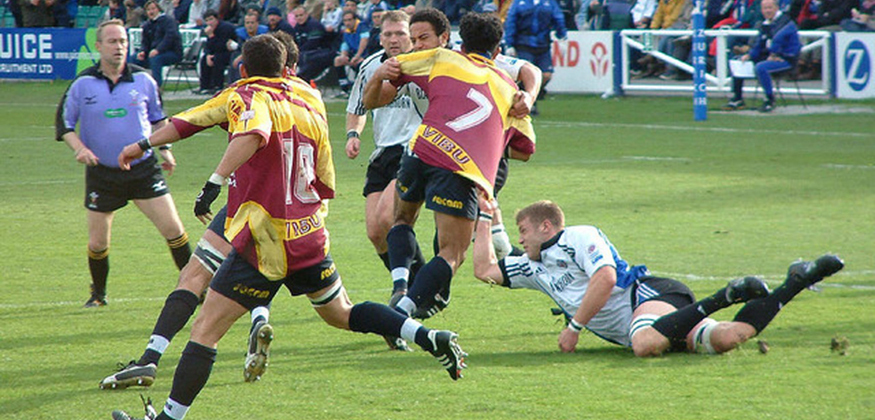#### Momentum in Everyday Life

###### February 12, 2019 Tom Clark| Comments OffIndoor games are getting popular across the globe due to their easy everyday access. Billiard is one of the most loved sports among the greatest population. Every day people try to come up with innovative ways to dispose billiard balls into pockets. In this process right movements of billiard balls are crucial. What actually governs these movements? The answer is simple: the momentum of one ball is transferred into another.

In general anything with the mass ‘m’ kgmoving with velocity ‘v’ m/sexert a momentum of ‘p’. Thus, the momentum can be defined as the product of mass and velocity for a single particle, mathematically written as Momentum
p=mv
The SI Unit of Momentum is kgm/s.The momentum has both magnitude and direction because it includes velocity. In short, momentum is a vector quantity. For example, in billiards game, the direction of the ball to be moved entirely depends on the initial position and direction of collision.

Similarly, when more than one particle is involved in the collision (For example when striker hits a group of coins during the carrom game) the total momentum is the vector sum of the momentum of individual particles.
p=p1+p2+p3+…+pn
=m1v!+m2v2+m3v3+⋯..+mnvn
Where pis the total momentum/resultant momentum and p1,p2,p3are the momentum of individual particles.

The concept of momentum is used in many real-life instances, starting from accidents between two vehicles to collision of astronomical objects. Thus, the famous Newton’s second law of motion is derived from the momentum. It states that “the net force acting on the body is directly proportional to the rate of change of momentum”.

Application of momentum is enormous. This classical mechanics concept forms a firm foundation for numerous advanced physics disciplines. To learn more such interesting concepts like, Electric Field, Magnetic Field, Electromagnets, Optics, Astrophysics, Nuclear Physics do visit our website and check the link given below to visualise the mechanism behind push and pull forces.